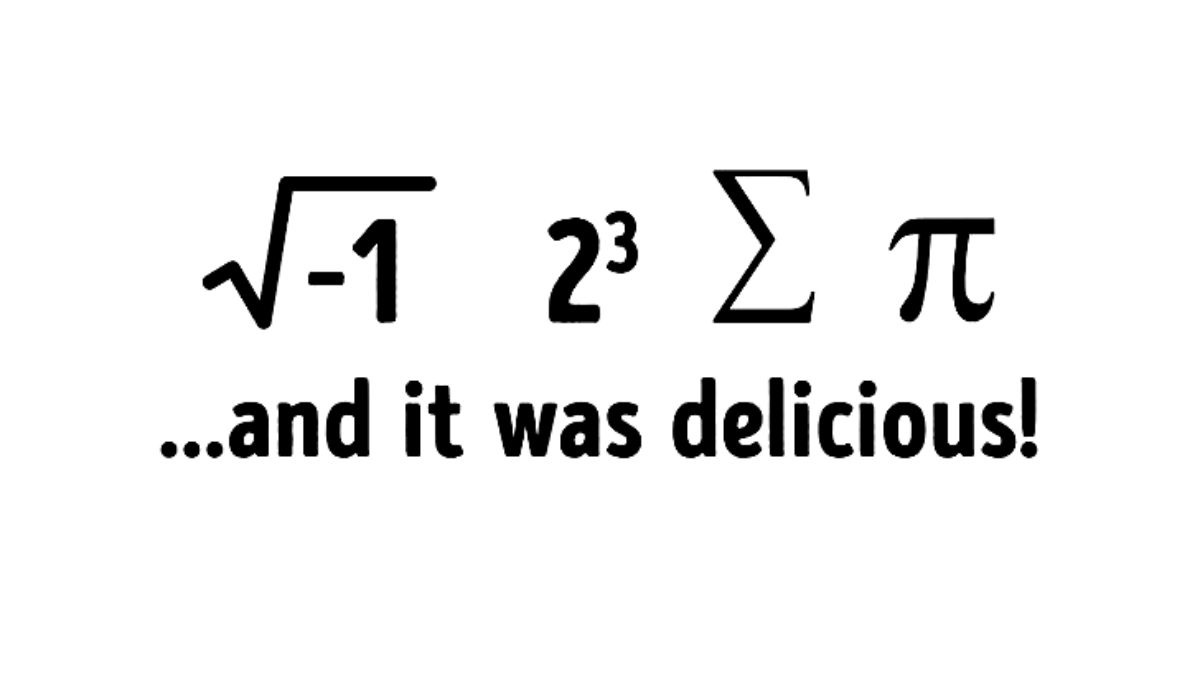# Brain Teaser Maths Puzzle: Can you spot words from Mathematical Numbers & Symbols in 7 secs?

Brain Teaser Maths Puzzle: In this brain riddle, you need to identify words from Mathematical Numbers Symbols and complete the sentence in the picture. Can you solve this puzzle in 7 seconds?Brain Teaser Maths Puzzle

Brain Teaser Maths Puzzle: If you like to solve riddles and puzzles in your leisure time, then this brain teaser is meant for you! Brain teasers can be considered as a trickier version of puzzles & riddles, as these brain games are solved with logical reasoning. For solving this type of quiz, you need to analyze the problem with little different and creative approach. You have to use a creative approach as the solution won’t be right in front of you. Similar to these lines, we have come up with an interesting brain teaser where you have to identify words from Mathematical Numbers & Symbols and complete the sentence in the image.

## Can you spot words from Mathematical Numbers & Symbols in 7 seconds?

Image Source: Bright Side

Can you identify the Ghost in the Room?

In the above image, you have to identify the words that are written in disguise of mathematical numbers and symbols. You need to replace number and symbols with four words and complete the sentence. The sentence will end with the words “… and it was delicious”. An active mind can solve this riddle within 7 seconds. You are required to look numbers and symbols carefully before answering the question as the answer is not as simple as you think. As a heads up, the answers to this brain teaser has been given right below the question, so make sure you don’t scroll too far and cheat!

Identify who is from the future in the 1970s picture

Hint: Identify the meaningful words for the mathematical numbers and symbols

In this brain puzzle, you need to complete the sentence by identifying the words that will replace the mathematical symbols and numbers in the picture. Also, these words should make a meaningful sentence when combined with the second half of the sentence. Let’s decode each mathematical numbers and symbols one by one:

Image Source: Bright Side

1. I-The first mathematical number under root minus one is also known as iota that explains complex numbers. The symbol for iota is ‘i’. So, the first word is ‘I’.
2. ATE - The second mathematical number is the cube of the number two (23) which is equal to 8. So, the second word will be ‘ate’.
3. SOME - The third mathematical symbol denotes the sum of all numbers. So, the second word will be ‘some’.
4. PIE - The fourth mathematical symbol denotes the number π which is a is a mathematical constant that is the ratio of a circle's circumference to its diameter. So, the fourth word will be ‘Pie’.

Can you spot who is Left-handed Person in the picture?

Using creative and analytical thinking will help you to derive answers in such brain teasers. This puzzle was a tricky but simple as it needs less time and brain power to solve. But it sure feels great when you figure one out the answer in few seconds.

Can you identify who is the thief in the picture?

Get the latest General Knowledge and Current Affairs from all over India and world for all competitive exams.
खेलें हर किस्म के रोमांच से भरपूर गेम्स सिर्फ़ जागरण प्ले पर